# Heights and Distances

Heights and Distances

In this topic, we will be studying some ways in which trigonometry is used in life around you.

What is meant by trigonometry?

Trigonometry is one of the most ancient subjects studied by scholars all over the world. Trigonometry was invented because its need arose in astronomy. Since then astronomers have used it, for instance, to calculate distances from the Earth to the planets and stars. Trigonometry is also used in geography and in navigation.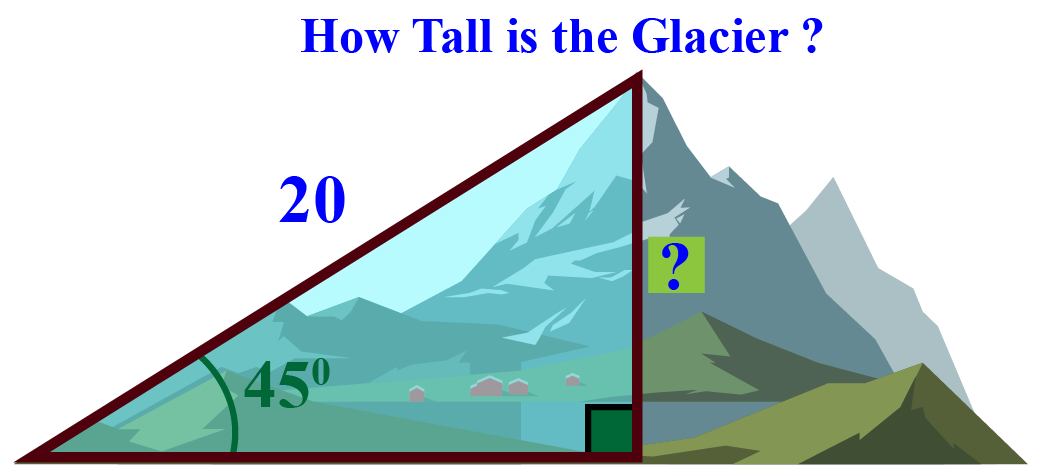The knowledge of trigonometry is used to find heights of structures, construct maps, determine the position of an island in relation to the longitudes and latitudes.

## Lesson Plan

 1 What Do You Mean by Heights and Distances? 2 Challenging Questions on Heights and Distances 3 Important Notes on Heights and Distances 4 Solved Examples on Heights and Distances 5 Interactive Questions on Heights and Distances

## What Do You Mean by Height and Distances?

The most significant definitions that are used when dealing with heights and distances are given as:

Line of sight: It is the line drawn from the eye of an observer to the point in the object viewed by the observer.

Here, the cat is the observer and the object is the bird.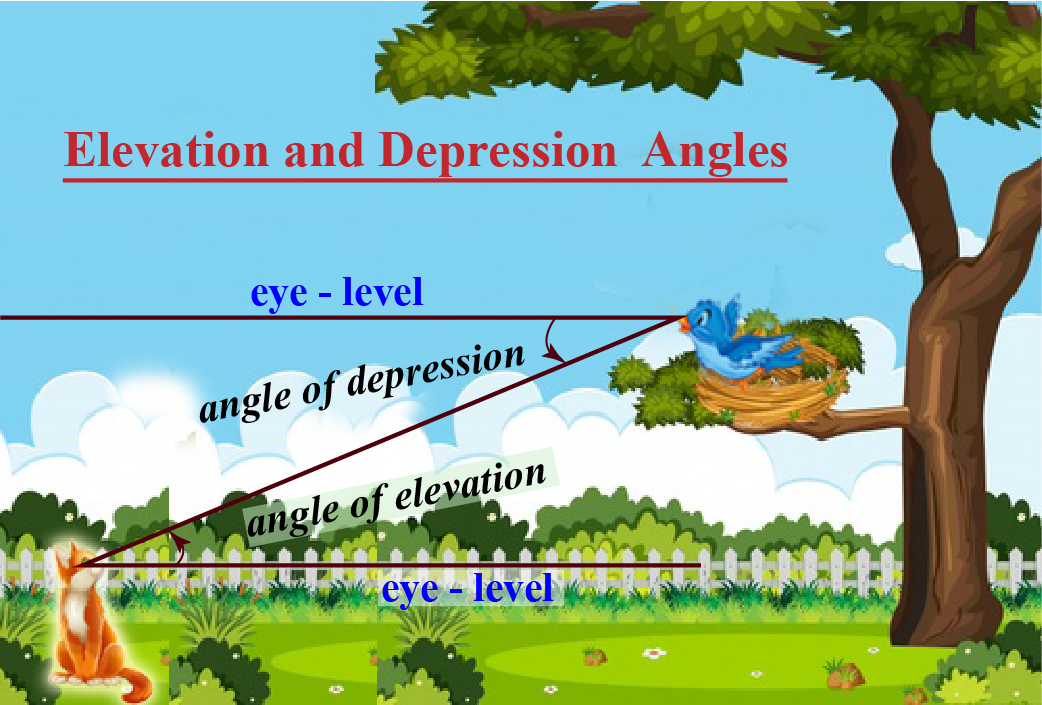The angle of elevation: The angle between the horizontal and the line of sight joining an observation point to an elevated object.

In the following figure, the angle of elevation of the kite from the point $$A$$ is $${30^\circ }$$, and from point $$B$$ is $${60^\circ }$$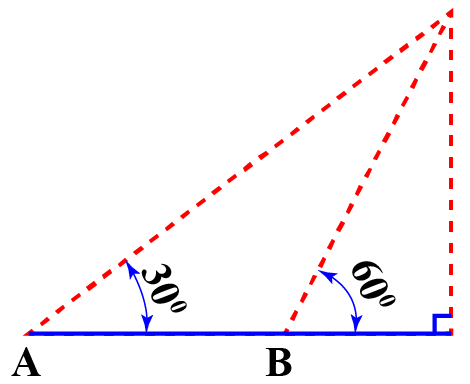The angle of depression: The angle between the horizontal and the line of sight joining an observation point to an object below the horizontal level.

In the following figure, if the top of the building is our observation point, then the angle of depression of person $$X$$ is $${45^\circ}$$, and that of person $$Y$$  is $${60^\circ }$$.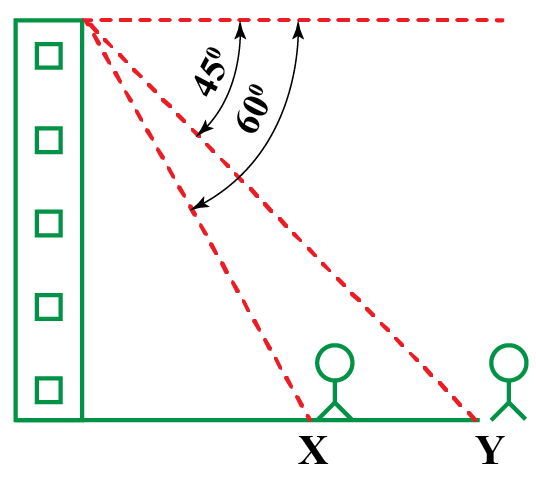Let us now experience how trigonometry is applied to practical situations.

## What Are the Formulas for Heights and Distances?

Trigonometric ratios can be used to find heights and distances. Some useful relations are illustrated by the below-given diagrams which help us to determine heights and distances.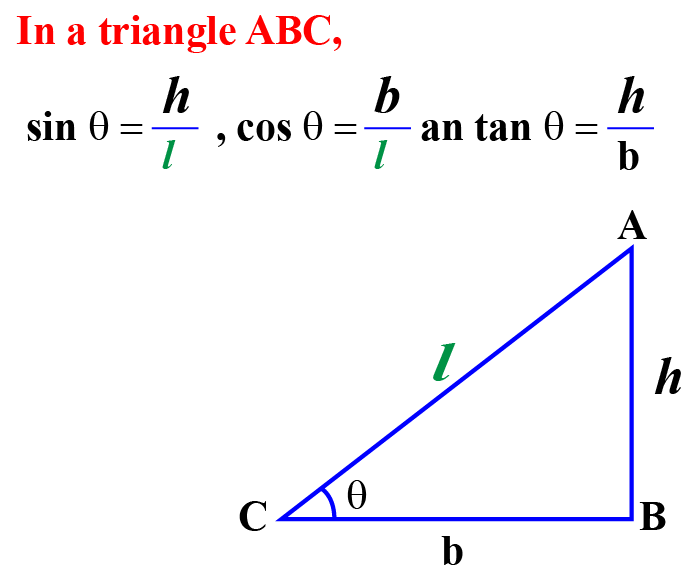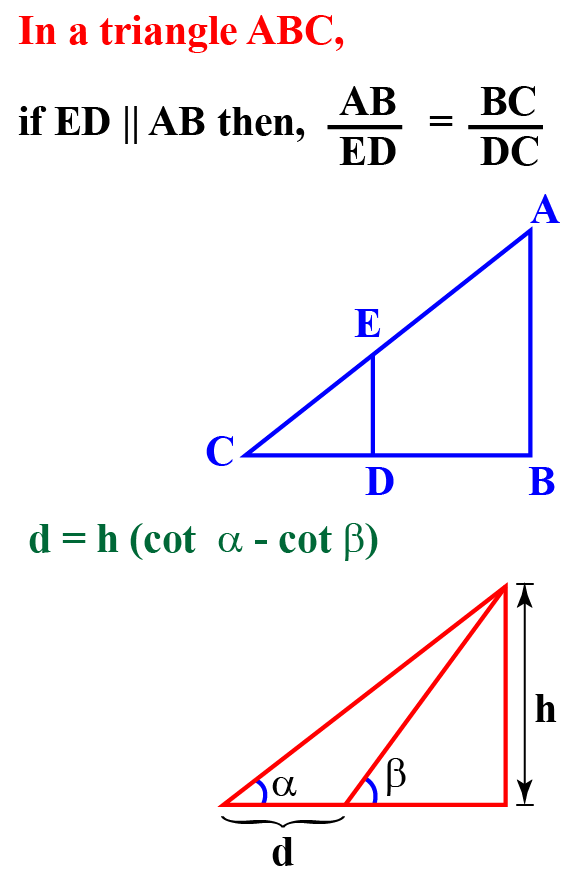Trigonometry
Trigonometry
Grade 9 | Questions Set 1
Trigonometry
Grade 10 | Questions Set 1
Trigonometry
More Important Topics
Numbers
Algebra
Geometry
Measurement
Money
Data
Trigonometry
Calculus
More Important Topics
Numbers
Algebra
Geometry
Measurement
Money
Data
Trigonometry
Calculus
Learn from the best math teachers and top your exams

• Live one on one classroom and doubt clearing
• Practice worksheets in and after class for conceptual clarity
• Personalized curriculum to keep up with school